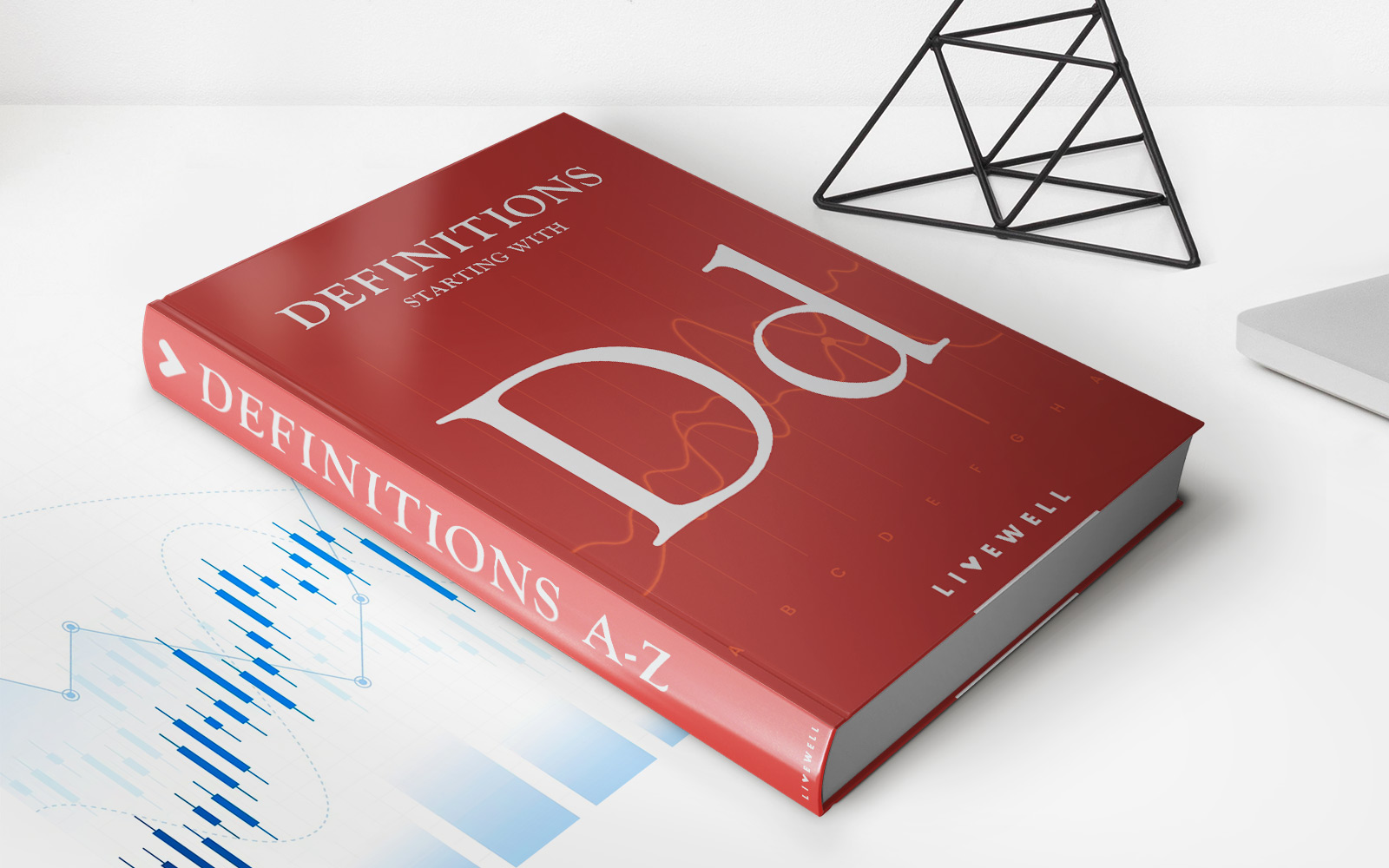Home>Finance>Depreciated Cost: Definition, Calculation Formula, ExampleFinance

# Depreciated Cost: Definition, Calculation Formula, Example

Learn the definition, calculation formula, and example of depreciated cost in finance. Gain a comprehensive understanding of this concept and its importance.

## Understanding Depreciated Cost in Finance

When it comes to managing your finances, understanding key concepts is essential. One of these important concepts is depreciated cost. In this article, we will explore the definition of depreciated cost, the formula for calculating it, and provide you with a real-life example to illustrate its application.

## Key Takeaways:

• Depreciated cost refers to the reduction in the value of an asset over time due to wear and tear, obsolescence, or other factors.
• Calculating depreciated cost involves subtracting the accumulated depreciation from the original cost of the asset.

## What is Depreciated Cost?

Depreciated cost is a financial concept that quantifies the decline in value of an asset over its useful life. In simple terms, it reflects how much an asset has depreciated or lost value since its purchase. Depreciation can occur due to various reasons such as normal wear and tear, technological advancements, or changes in market demands.

Depreciation is particularly relevant when it comes to accounting for fixed assets like buildings, machinery, or vehicles. By calculating the depreciated cost, businesses can accurately reflect the reduced value of their assets and better understand their overall financial standing.

## Calculating Depreciated Cost: The Formula

To calculate the depreciated cost of an asset, you need two key pieces of information: the original cost of the asset and the accumulated depreciation. The formula for calculating depreciated cost is as follows:

Depreciated Cost = Original Cost – Accumulated Depreciation

Let’s break down the formula:

1. Original Cost: This is the initial cost at which the asset was acquired. It includes the purchase price as well as any additional costs incurred to bring the asset into working condition.
2. Accumulated Depreciation: This represents the total depreciation incurred on the asset over its useful life. It takes into account the passage of time, wear and tear, and any other relevant factors.

By subtracting the accumulated depreciation from the original cost, you arrive at the depreciated cost, which reflects the asset’s current value.

## Example of Depreciated Cost Calculation

Let’s consider an example to illustrate how to calculate the depreciated cost. Suppose a business purchases a delivery truck for \$50,000. The estimated useful life of the truck is 5 years, with no estimated residual value at the end of its lifespan. Using the straight-line depreciation method, the business applies an equal amount of depreciation each year.

To calculate the annual depreciation, divide the original cost by the useful life: \$50,000 / 5 = \$10,000. Therefore, each year, the truck depreciates by \$10,000.

After 3 years, the accumulated depreciation on the truck would be: \$10,000 x 3 = \$30,000. Therefore, the depreciated cost of the truck at that point would be: \$50,000 – \$30,000 = \$20,000.

So, after 3 years, the truck’s depreciated cost would be \$20,000.

## Conclusion

Depreciated cost plays a crucial role in financial management, enabling businesses to accurately assess the value of their assets. By understanding the definition, formula, and calculation process, you can make informed decisions regarding asset management and financial planning. Remember, depreciated cost reflects the tangible decline in an asset’s value over time, offering valuable insights into the overall financial health of a business.

Stay tuned for more articles on various finance topics to help you further navigate the world of personal and business finance!

### Related Post

• https://livewell.com/finance/depreciated-cost-definition-calculation-formula-example/